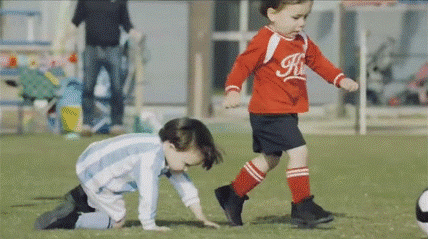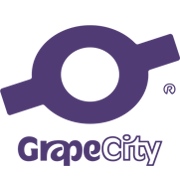三道简单算法题(二)

+关注继续查看

1：试着用最少的比较次数去寻找数组中的最大值和最小值。

//1：试着用最少的比较次数去寻找数组中的最大值和最小值。
void FindMaxMin(int *A,int size,int* Max,int* Min)
{
int i=(size & 1)?1:0;
*Max=*Min=A;
for(;i<size;i=i+2)
{
if(A[i]>A[i+1])
{
if(A[i]>*Max)
*Max=A[i];
if(A[i+1]<*Min)
*Min=A[i+1];
}else{
if(A[i+1]>*Max)
*Max=A[i+1];
if(A[i]<*Min)
*Min=A[i];
}
}
}

void FindMaxMinTest(){
int A={2,4,6,8,9,1,3,5,7};
int Max=0;
int Min=0;
FindMaxMin(A,9,&Max,&Min);
cout<<Max<<endl;
cout<<Min<<endl;
}

2：给一个整数数组，求数组中重复出现次数大于数组总个数一半的数

//2：给一个整数数组，求数组中重复出现次数大于数组总个数一半的数
int MoreThanHalf(int *A,int size)
{
int num=A;
int count=1;
for(int i=1;i<size;i++)
{
if(num==A[i])
{
count++;
}else{
count--;
if(count==0)
{
num=A[i];
count=1;
}
}
}

count=0;
for(int i=0;i<size;i++)
{
if(A[i]==num)
count++;
}
return count>(size/2)? num:-1;
}

void MoreThanHalfTest()
{
int A={ 1, 1,2, 1, 2, 2, 1, 2, 2 };
cout<<MoreThanHalf( A,9);
}

3：给一个很大的数组，里面有两个数只出现过一次，其他数都出现过两次，把这两个数找出来

//3：给一个很大的数组，里面有两个数只出现过一次，其他数都出现过两次，把这两个数找出来
void FindTwoNum(int* A,int size, int* first,int* second)
{
int excVal=A;
for(int i=1;i<size;i++)
{
excVal^=A[i];
}
int s1=1;
int s2=excVal;
while((s2&1)==0)
{
s2>>=1;
s1<<=1;
}
*first=excVal;
for(int i=0;i<size;i++)
{
if(s1&A[i])
*first^=A[i];
}
*second=excVal^*first;
}

void FindTwoNumTest()
{
int A={2,1,1,2,3,3,5,7,4,4,7,9};
int first=0,second=0;
FindTwoNum(A,12,&first,&second);
cout<<first<<endl;
cout<<second;
}9998 0RSA与AES混合加密算法的实现
RSA与AES加密算法所产生的密钥数不一样，它们是如何进行加密的呢？ 接收方生成RSA密钥对，将其中的RSA公钥传递给发送方(接收方与发送方建立连接是需要认证的，SSL/TLS协议可以确保RSA公钥的安全完整)，然后用RSA公钥对AES密钥进行加密，加密后的结果传递给接收方，接收方用RSA私钥解密后，得到AES密钥，最后使用AES密钥解密，从而达到安全互通数据的目的。(如下图所示)
1569 010880 0RANSAC算法在图像拼接上的应用的实现

2290 0751 013793 011879 0Silverlight中非对称加密及数字签名RSA算法的实现
RSA算法是第一个既能用于数据加密也能用于数字签名的算法。它易于理解和操作，也很流行。它的安全性是基于大整数素因子分解的困难性，而大整数因子分解问题是数学上的著名难题，至今没有有效的方法予以解决，因此可以确保RSA算法的安全性。
669 01185 07327 0
1703

0

《2021云上架构与运维峰会演讲合集》

《零基础CSS入门教程》

《零基础HTML入门教程》